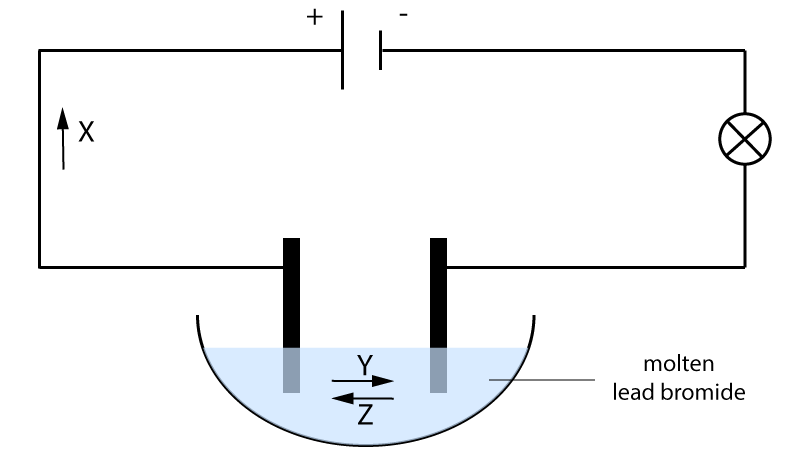Chemistry Edexcel iGCSE:
Electrolysis

Page 1

Edexcel triple science only (not for double science students).

1. Which of the following statements about conduction of electricity by compounds are true?

 I. All compounds conduct electricity when molten or aqueous II. Covalent compounds do not conduct in any state III. Ionic compounds do not conduct when solid

• A.   I and II only
• B.   I and III only
• C.   II and III only
• D.   I, II and III
2. Which statement about the conduction of electricity by compounds is true?
• A.   Covalent compounds conduct when liquid as molecules are free to move
• B.   Ionic compounds conduct in aqueous solution as ions are free to move
• C.   Covalent compounds do not conduct when solid as ions are not free to move
• D.   Ionic compounds conduct when molten as electrons are free to move

3. Which is true about the ion attracted to the cathode?

 Type of ion Charge on ion A anion positive B anion negative C cation positive D cation negative

Q4-7:
The diagram shows the apparatus used to electrolyse lead(II)bromide. The wires connected to the electrodes are made from copper. During electrolysis of the molten lead(II)bromide particles X , Y and Z move in the directions shown by the arrows.4. Electrolysis does not occur until the lead(II)bromide is molten because when solid the ..
• A.   electrons are not free to move
• B.   electrons are free to move
• C.   ions are not free to move
• D.   ions are free to move
5. Particle X is a .
6. Particle Y is a .
7. Particle Z is a .

Q8-9:
Magnesium is extracted by electrolysis of molten magnesium chloride (MgCl2) obtained from sea water.

8. The half equation for the reaction occurring at the positive electrode is …

• A.   Cl-  Cl + e-
• B.   2Cl-  Cl2 + 2e-
• C.   Cl- + e-  Cl
• D.   2Cl- + 2e- Cl2

9.The half-equation for the reaction occurring at the negative electrode and the type of reaction occurring is:

 Half-equation Type of reaction A Mg2+ → Mg + 2e- oxidation B Mg2+ → Mg + 2e- reduction C Mg2+ + 2e- → Mg oxidation D Mg2+ + 2e- → Mg reduction